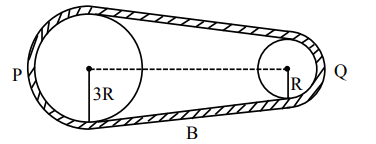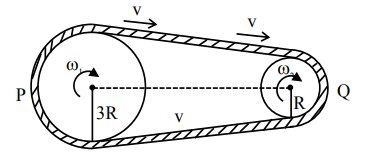# In the given figure, two wheels P and Q are connected by a belt B. The radius of P is three times as that of Q.`
Question:

In the given figure, two wheels P and Q are connected by a belt B. The radius of P is three times as that of Q. In case of same rotational

kinetic energy, the ratio of rotational inertias $\left(\frac{\mathrm{I}_{1}}{\mathrm{I}_{2}}\right)$

will be x : 1. The value of x will be _____.Solution:$\frac{1}{2} \mathrm{I}_{1}\left(\omega_{1}\right)^{2}=\frac{1}{2} \mathrm{I}_{2}\left(\omega_{2}\right)^{2}$

$\mathrm{I}_{1}\left(\frac{\mathrm{v}}{3 \mathrm{R}}\right)^{2}=\mathrm{I}_{2}\left(\frac{\mathrm{v}}{\mathrm{R}}\right)^{2}$

$\frac{\mathrm{I}_{1}}{\mathrm{I}_{2}}=\frac{9}{1}$